# Using Tables toto Explore Using Tables Explore 7

• Slides: 19Using Tables toto Explore Using Tables Explore 7 -2 Equivalent Ratios and Rates Warm Up Problem of the Day Lesson Presentation Course 11Using Tables to Explore 7 -2 Equivalent Ratios and Rates Warm Up Find the unit rate. 1. 16 miles in 4 hours 4 mi/h 2. 3 oranges for \$2. 40 \$0. 80 per orange 3. 3 bottles for \$0. 93 \$0. 31 per bottle 4. 6 DVDs for \$36. 60 \$6. 10 per DVD Course 1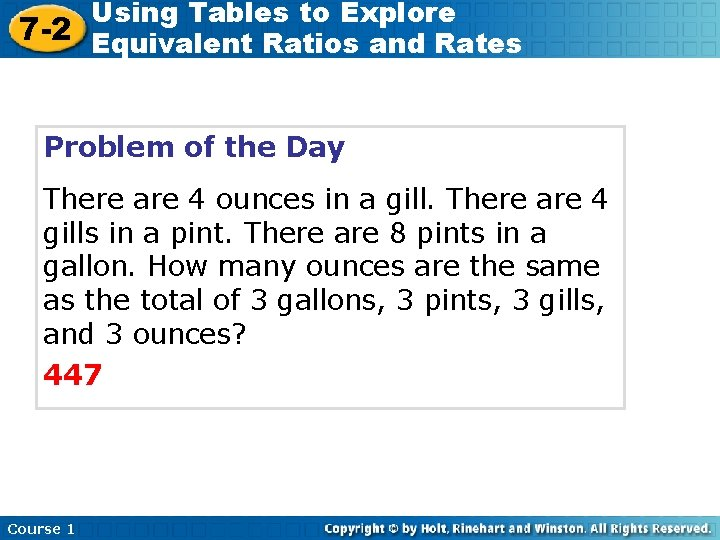Using Tables to Explore 7 -2 Equivalent Ratios and Rates Problem of the Day There are 4 ounces in a gill. There are 4 gills in a pint. There are 8 pints in a gallon. How many ounces are the same as the total of 3 gallons, 3 pints, 3 gills, and 3 ounces? 447 Course 1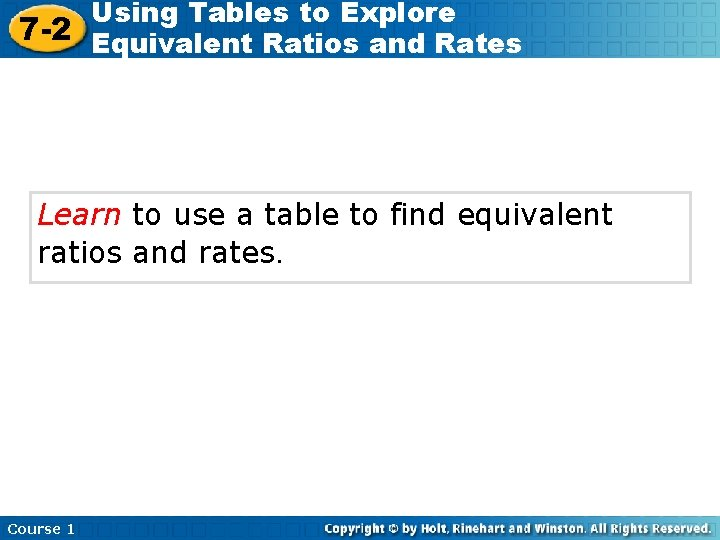Using Tables to Explore 7 -2 Equivalent Ratios and Rates Learn to use a table to find equivalent ratios and rates. Course 1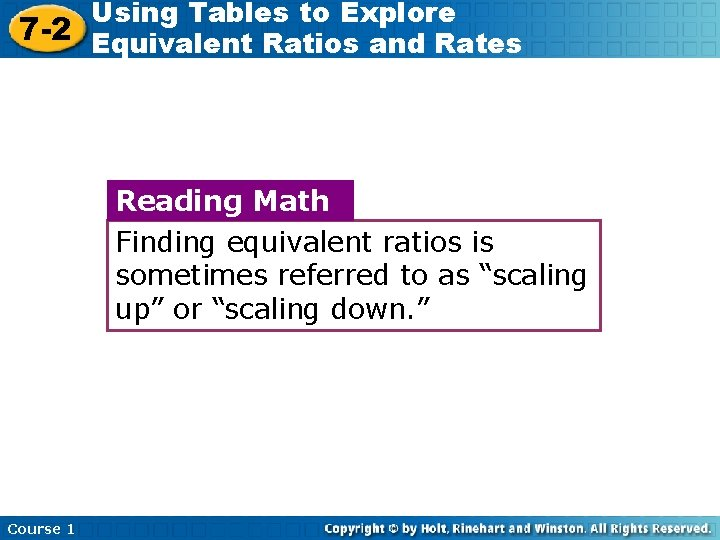Using Tables to Explore 7 -2 Equivalent Ratios and Rates Reading Math Finding equivalent ratios is sometimes referred to as “scaling up” or “scaling down. ” Course 1Using Tables to Explore 7 -2 Equivalent Ratios and Rates Use a table to find ratios equivalent to 3 to 2. Original 3 Ratio • 2 3 • 3 3 • 4 Pints of yellow 3 6 9 12 Pints of blue 2 4 6 8 2 • 2 2 • 3 2 • 4 You can increase amounts but keep them in the same ratio by multiplying both the numerator and denominator of the ratio by the same number. The ratios 3 to 2, 6 to 4, 9 to 6, and 12 to 8 are equivalent. You can also decrease amounts in the same ratio by dividing the numerator and denominator by the same number. Course 1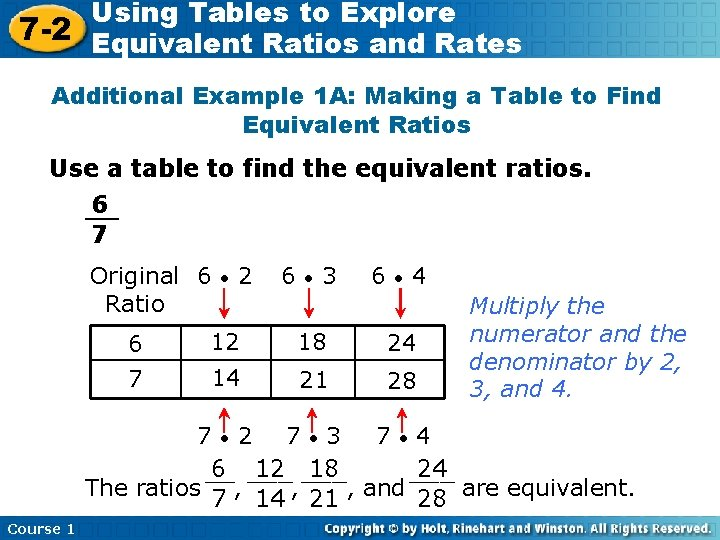Using Tables to Explore 7 -2 Equivalent Ratios and Rates Additional Example 1 A: Making a Table to Find Equivalent Ratios Use a table to find the equivalent ratios. 6 __ 7 Original 6 Ratio • 2 6 • 3 6 • 4 6 12 18 24 7 14 21 28 Multiply the numerator and the denominator by 2, 3, and 4. 7 • 2 7 • 3 7 • 4 6 ___ 12 ___ 18 24 __ ___ The ratios , 7 14 , 21 , and 28 are equivalent. Course 1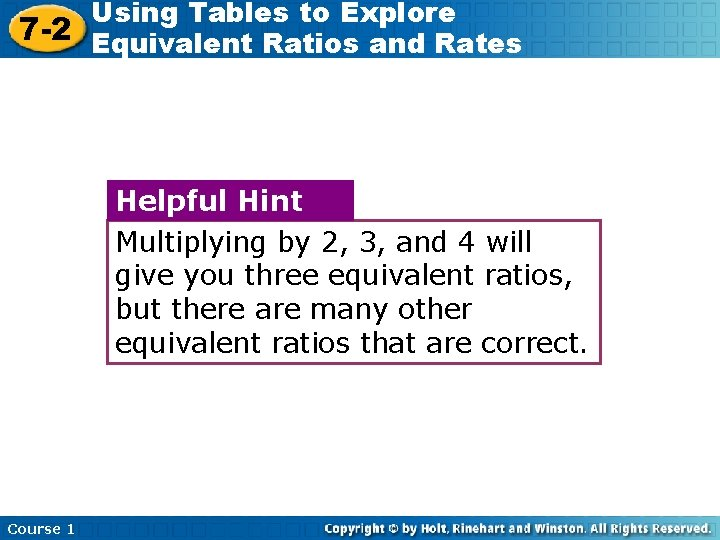Using Tables to Explore 7 -2 Equivalent Ratios and Rates Helpful Hint Multiplying by 2, 3, and 4 will give you three equivalent ratios, but there are many other equivalent ratios that are correct. Course 1Using Tables to Explore 7 -2 Equivalent Ratios and Rates Additional Example 1 B: Making a Table to Find Equivalent Ratios Use a table to find the equivalent ratios. 3 to 5 Original 3 Ratio • 2 3 • 3 3 • 4 3 6 9 12 5 10 15 20 5 • 2 5 • 3 5 • Multiply the numerator and the denominator by 2, 3, and 4. 4 The ratios 3 to 5, 6 to 10, 9 to 15, and 12 to 20 are equivalent. Course 1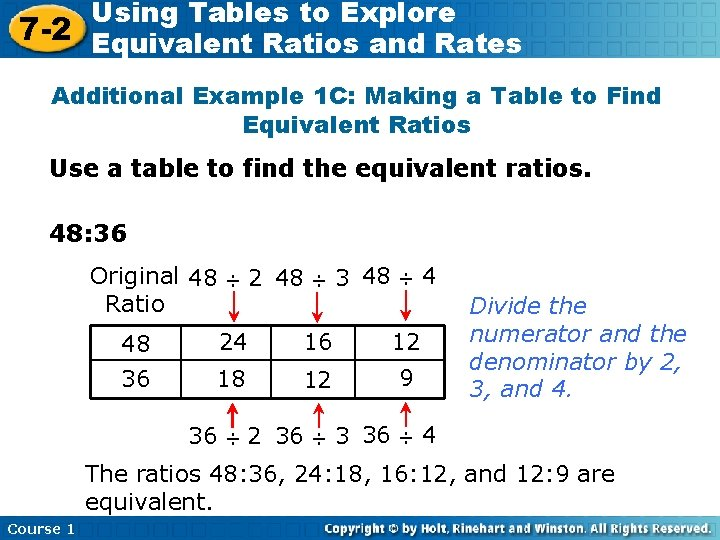Using Tables to Explore 7 -2 Equivalent Ratios and Rates Additional Example 1 C: Making a Table to Find Equivalent Ratios Use a table to find the equivalent ratios. 48: 36 Original 48 Ratio 2 48 ÷ ÷ 3 48 ÷ 48 24 16 12 36 18 12 9 36 ÷ 2 36 ÷ 3 36 ÷ 4 Divide the numerator and the denominator by 2, 3, and 4. 4 The ratios 48: 36, 24: 18, 16: 12, and 12: 9 are equivalent. Course 1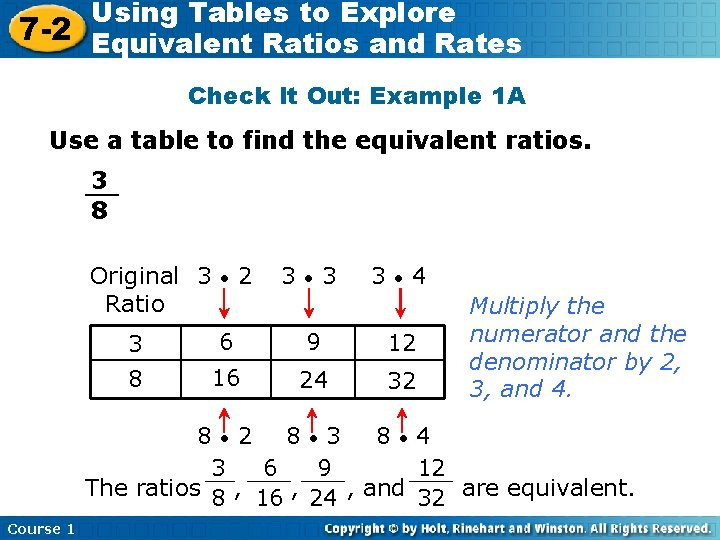Using Tables to Explore 7 -2 Equivalent Ratios and Rates Check It Out: Example 1 A Use a table to find the equivalent ratios. 3 __ 8 Original 3 Ratio • 2 3 • 3 3 • 4 3 6 9 12 8 16 24 32 Multiply the numerator and the denominator by 2, 3, and 4. 8 • 2 8 • 3 8 • 4 3 ___ 6 ___ 9 12 __ ___ The ratios 8 , 16 , 24 , and 32 are equivalent. Course 1Using Tables to Explore 7 -2 Equivalent Ratios and Rates Check It Out: Example 1 B Use a table to find the equivalent ratios. 2 to 9 Original 2 Ratio • 2 2 • 3 2 4 6 9 18 27 9 • 2 • 4 Multiply the numerator and the denominator by 2, 3, and 4. 8 36 3 9 • 4 The ratios 2 to 9, 4 to 18, 6 to 27, and 8 to 36 are equivalent. Course 1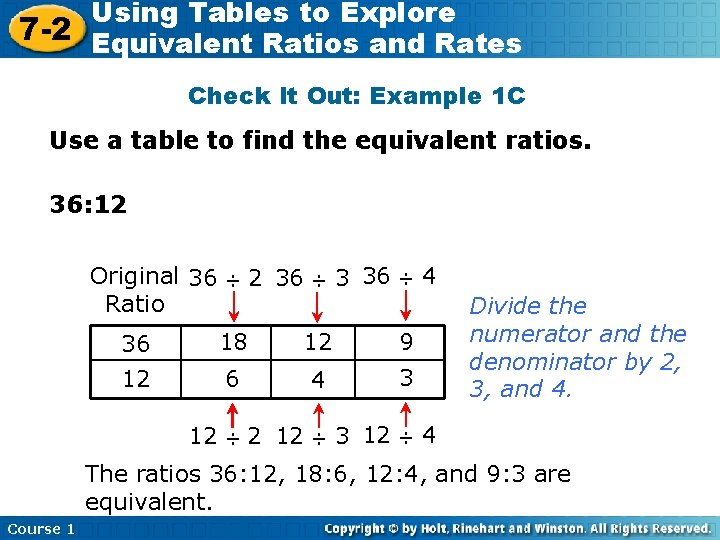Using Tables to Explore 7 -2 Equivalent Ratios and Rates Check It Out: Example 1 C Use a table to find the equivalent ratios. 36: 12 Original 36 Ratio 2 36 ÷ ÷ 3 36 ÷ 36 18 12 9 12 6 4 3 12 ÷ 2 12 ÷ 3 12 ÷ 4 Divide the numerator and the denominator by 2, 3, and 4. 4 The ratios 36: 12, 18: 6, 12: 4, and 9: 3 are equivalent. Course 1Using Tables to Explore 7 -2 Equivalent Ratios and Rates Additional Example 2: Application Several groups of friends are going to take a shuttle bus to the park. The table shows how much the different groups will pay in all. Predict how much a group of 15 friends will pay. Number in Group Bus Fare(\$) 6 12 12 24 18 36 12< 15 < 18; therefore, the group will pay between \$24 and \$36. Course 1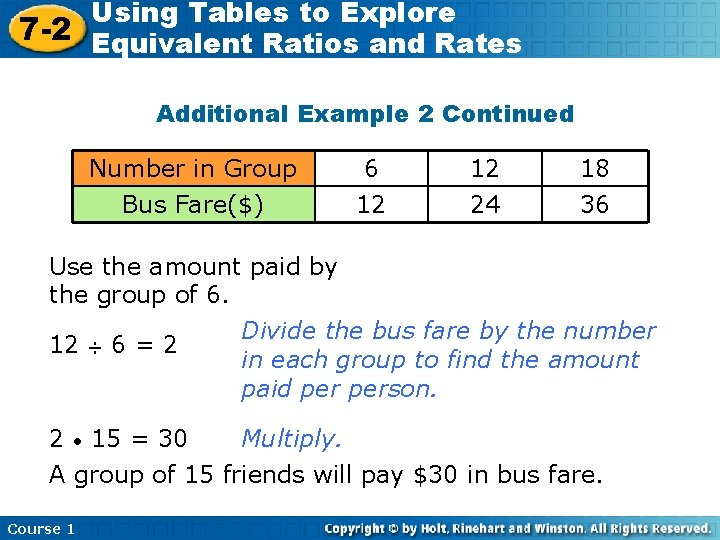Using Tables to Explore 7 -2 Equivalent Ratios and Rates Additional Example 2 Continued Number in Group Bus Fare(\$) 6 12 12 24 18 36 Use the amount paid by the group of 6. Divide the bus fare by the number 12 ÷ 6 = 2 in each group to find the amount paid person. 2 • 15 = 30 Multiply. A group of 15 friends will pay \$30 in bus fare. Course 1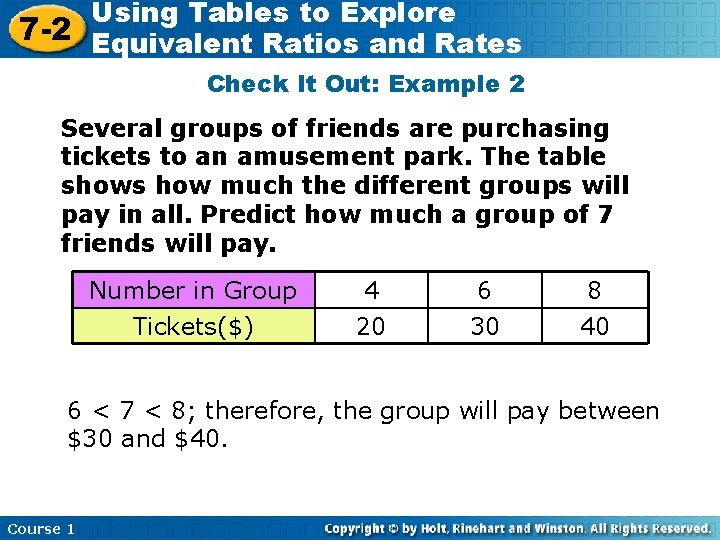Using Tables to Explore 7 -2 Equivalent Ratios and Rates Check It Out: Example 2 Several groups of friends are purchasing tickets to an amusement park. The table shows how much the different groups will pay in all. Predict how much a group of 7 friends will pay. Number in Group Tickets(\$) 4 20 6 30 8 40 6 < 7 < 8; therefore, the group will pay between \$30 and \$40. Course 1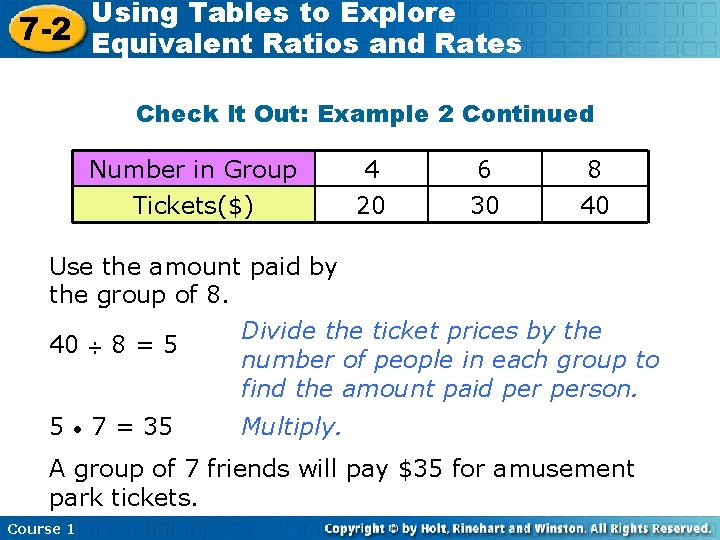Using Tables to Explore 7 -2 Equivalent Ratios and Rates Check It Out: Example 2 Continued Number in Group Tickets(\$) 4 20 6 30 8 40 Use the amount paid by the group of 8. Divide the ticket prices by the 40 ÷ 8 = 5 number of people in each group to find the amount paid person. 5 • 7 = 35 Multiply. A group of 7 friends will pay \$35 for amusement park tickets. Course 1Using Tables to Explore 7 -2 Equivalent Ratios and Rates Lesson Quiz: Part I Use a table to find three equivalent ratios. Possible Answers: 1 __ 1. 3 2 , __ 3 , __ 4 __ 6 9 12 12 3. __ 60 6 , __ 2 , __ 1 __ 30 10 5 Course 1 9 __ 2. 4 18 , __ 27 , __ 36 __ 8 12 16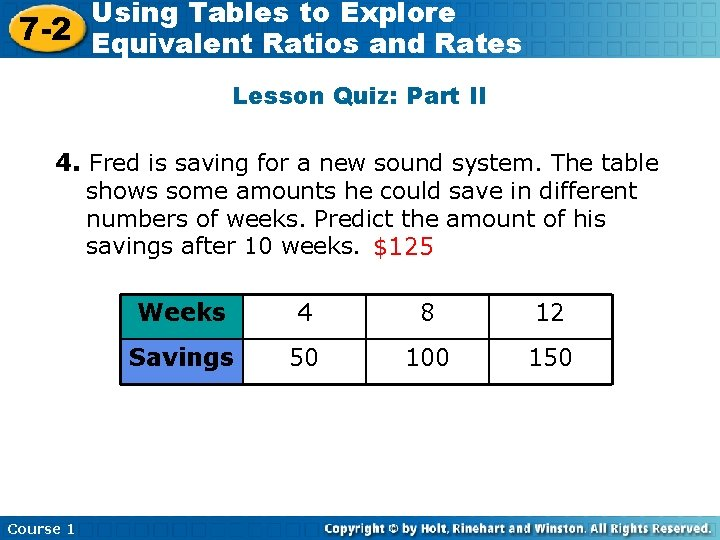Using Tables to Explore 7 -2 Equivalent Ratios and Rates Lesson Quiz: Part II 4. Fred is saving for a new sound system. The table shows some amounts he could save in different numbers of weeks. Predict the amount of his savings after 10 weeks. \$125 Course 1 Weeks 4 8 12 Savings 50 100 150